Next: Results and Discussion Up: Evolution of Supernova Explosion Previous: - Dependence for the

##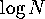-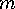for Supernova Explosions

In order to construct the-dependence for supernova explosions, we calculated separately the m(z) in the R band and N(z) taking the cosmological and evolutionary effects into account. Thus we get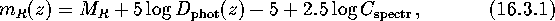where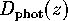is the photometric distance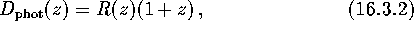andis the spectral correctionwhereis the spectral distribution of the energy flux from a supernova and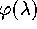is the filter transmission function.

We have not taken extinction (both inside galaxies and en routeto Earth) into account, although of course it will affect the observed m(z) and N(z). It is especially important for SN of type II because they are usually located in star-forming regions rich in dust and gas; this may considerably alter the observed magnitude and even make detection impossible.

We calculate thedependence for supernovae at the maximum brightness, assuming the SN spectrum to be black body with a temperature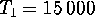ĀK for SN Ia,ĀK for SN II and SN Ib, and M(R) at maximum be -18.9, -17.3 and -16.3 for SN Ia, SN Ib and SN II, respectively (van den Bergh and Tammann, 1991). The Hubble constant was taken to be H=75Ākm sMpc.

Although the observed ultraviolet spectra of SN I is much weaker than the approximated black body law, we can still use this approximation for the R band up to the redshifts of, whereis the characteristic wavelength of the ultraviolet ``drop'' in the spectra. For larger z, the effect of the ultraviolet emission suppression in SN I spectra would result in an almost complete lack of SN I fainter than some limit. The dependence of m on z is displayed in FigureĀ52.

ĀFigure 52: The m(z) dependence for various supernova types.Ā

The number of SN per unit time within the sphere of redshift less than z is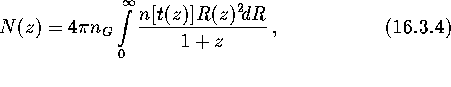where n(t) is the SN rate as a function of age of the galaxy, t(z) is the age of the galaxy as a function of its redshift, and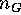is the density of galaxies in the Universe. We assumedĀMpc, which corresponds to the fractional density of stars relative to the critical density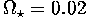.

We use a conventional cosmological model of a flat Universe without cosmological constant(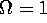,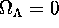). In this case,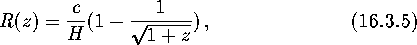and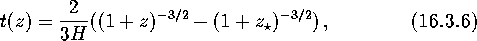where c is the velocity of light, andis the redshift of the initial star formation.

We assumed that the SN spectrum and luminosity are both independent of the age of the galaxy, and that the SN rate evolution n(t) significantly influences the-law.Next: Results and Discussion Up: Evolution of Supernova Explosion Previous: - Dependence for the

Mike E. Prokhorov
Sat Feb 22 18:38:13 MSK 1997